## GeoPandas的用法

### 读取空间数据

geopandas可以读取任意基于矢量的空间数据格式，包括ESRI shapefile，GeoJSON文件等命令：

``````gpd.read_file()

``````

In :
```import fiona
```

``help(fiona.open)``

### 写入空间数据

GeoDataFrames可以使用GeoDataFrame.to_file（）方法导出许多不同的标准格式。若查看支持的完整格式列表，请键入import fiona; fiona.supported_drivers。

### 地图工具

geopandas提供了一个用于制作地图的高级接口matplotlib库。其映射形状与GeoSeries或GeoDataFrame使用plot（）方法一样便于操作。

In :
```%matplotlib inline
import geopandas as gpd

```

In :
```# Examine country GeoDataFrame
```
Out:
continent gdp_md_est geometry iso_a3 name pop_est
0 Asia 22270.0 POLYGON ((61.21081709172574 35.65007233330923,... AFG Afghanistan 28400000.0
1 Africa 110300.0 (POLYGON ((16.32652835456705 -5.87747039146621... AGO Angola 12799293.0
2 Europe 21810.0 POLYGON ((20.59024743010491 41.85540416113361,... ALB Albania 3639453.0
3 Asia 184300.0 POLYGON ((51.57951867046327 24.24549713795111,... ARE United Arab Emirates 4798491.0
4 South America 573900.0 (POLYGON ((-65.50000000000003 -55.199999999999... ARG Argentina 40913584.0
In :
``` world.plot();
```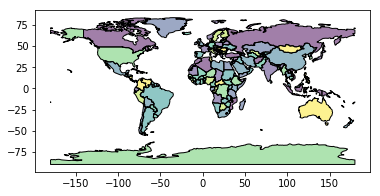#### Chloropleth地图

geopandas创建Chloropleth地图比较简单（每个形状颜色对应于相关联变量值的地图）。只需使用plot命令，将列参数设置为要用于分配颜色值的列即可。

In :
```# Plot by GDP per capta
world = world[(world.pop_est>0) & (world.name!="Antarctica")]

world['gdp_per_cap'] = world.gdp_md_est / world.pop_est

world.plot(column='gdp_per_cap');
```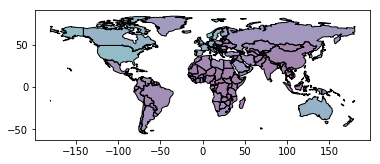In :
```world.plot(column='gdp_per_cap', cmap='OrRd');
```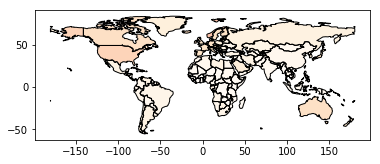In :
```#world.plot(column='gdp_per_cap', cmap='OrRd', scheme='quantiles');
```

#### 地图图层

In :
```# Look at capitals
# Note use of standard `pyplot` line style options
cities.plot(marker='*', color='green', markersize=5);

# Check crs
cities = cities.to_crs(world.crs)

# Now we can overlay over country outlines
# And yes, there are lots of island capitals
# apparently in the middle of the ocean!
```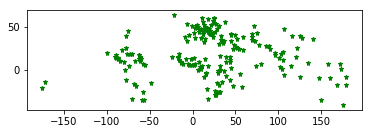In :
```base = world.plot(color='white')

cities.plot(ax=base, marker='o', color='red', markersize=5);
```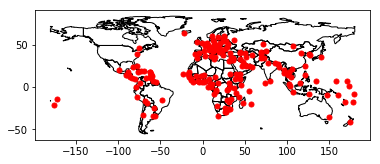In :
```import matplotlib.pyplot as plt

fig, ax = plt.subplots()

# set aspect to equal. This is done automatically
# when using *geopandas* plot on it's own, but not when
# working with pyplot directly.
ax.set_aspect('equal')

world.plot(ax=ax, color='white')
```
Out:
`<matplotlib.axes._subplots.AxesSubplot at 0x7f60cb38d278>`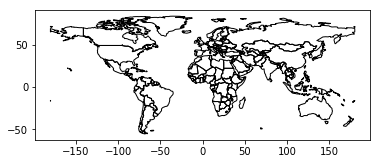In :
```cities.plot(ax=ax, marker='o', color='red', markersize=5)
```
Out:
`<matplotlib.axes._subplots.AxesSubplot at 0x7f60cb38d278>`
`<matplotlib.figure.Figure at 0x7f60cb388278>`
In :
```fig, ax = plt.subplots()

# set aspect to equal. This is done automatically
# when using *geopandas* plot on it's own, but not when
# working with pyplot directly.
ax.set_aspect('equal')

world.plot(ax=ax, color='white')

cities.plot(ax=ax, marker='o', color='red', markersize=5)

plt.show();
```#### 管理投影

1.坐标参考系统

CRS非常重要，其意义在于GeoSeries或GeoDataFrame对象中的几何形状是任意空间中的坐标集合。 CRS可以使Python的坐标与地球上的具体实物点相关联。

``````"+proj=longlat +ellps=WGS84 +datum=WGS84 +no_defs"

``````

``````"+init=epsg:4326".

``````

geopandas包含有CRS的表示方法，包括proj4字符串本身

`````` ("+proj=longlat +ellps=WGS84 +datum=WGS84 +no_defs")

``````

``````{'proj': 'latlong', 'ellps': 'WGS84', 'datum': 'WGS84', 'no_defs': True}

``````

• WGS84纬度/经度：“+ proj = longlat + ellps = WGS84 + datum = WGS84 + no_defs”或“+ init = epsg：4326”
• UTM区域（北）：“+ proj = utm + zone = 33 + ellps = WGS84 + datum = WGS84 + units = m + no_defs”
• UTM Zones（南）：“+ proj = utm + zone = 33 + ellps = WGS84 + datum = WGS84 + units = m + no_defs + south”

2.设置投影

``````my_geoseries.crs = {'init' :'epsg:4326'}

``````

3.重新投影

In :
```# load example data

# Check original projection
# (it's Platte Carre! x-y are long and lat)
world.crs
```
Out:
`{'init': 'epsg:4326'}`
In :
```# Visualize
world.plot();
```In :
```# Reproject to Mercator (after dropping Antartica)
world = world[(world.name != "Antarctica") & (world.name != "Fr. S. Antarctic Lands")]
world = world.to_crs({'init': 'epsg:3395'}) # world.to_crs(epsg=3395) would also work
world.plot();
```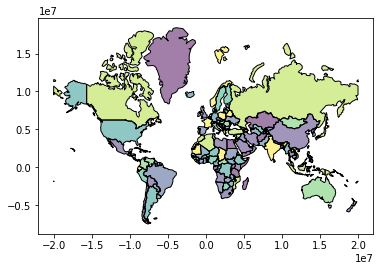### 五、几何操作

geopandas在shapely库中提供了所有几何操作的工具。

#### 1、创建方法

``````GeoSeries.buffer(distance, resolution=16)

``````

``````GeoSeries.boundary

``````

``````GeoSeries.centroid

``````

``````GeoSeries.convex_hull

``````

``````GeoSeries.envelope

``````

``````GeoSeries.simplify(tolerance, preserve_topology=True)

``````

``````GeoSeries.unary_union

``````

#### 2、仿射变换

``````GeoSeries.rotate(self, angle, origin='center', use_radians=False)

``````

``````GeoSeries.scale(self, xfact=1.0, yfact=1.0, zfact=1.0, origin='center')

``````

``````GeoSeries.skew(self, angle, origin='center', use_radians=False)

``````

``````GeoSeries.translate(self, angle, origin='center', use_radians=False)

``````

#### 3、几何操作的示例

In :
```%matplotlib inline

import geopandas as gpd
from geopandas import GeoDataFrame
from shapely.geometry import Polygon
from geopandas import GeoSeries
p1 = Polygon([(0, 0), (1, 0), (1, 1)])
p2 = Polygon([(0, 0), (1, 0), (1, 1), (0, 1)])
p3 = Polygon([(2, 0), (3, 0), (3, 1), (2, 1)])
g = GeoSeries([p1, p2, p3])

```

In :
```print (g.area)
```
```0    0.5
1    1.0
2    1.0
dtype: float64
```

In :
```g.buffer(0.5)
```
Out:
```0    POLYGON ((-0.3535533905932737 0.35355339059327...
1    POLYGON ((-0.5 0, -0.5 1, -0.4975923633360985 ...
2    POLYGON ((1.5 0, 1.5 1, 1.502407636663901 1.04...
dtype: object```

GeoPandas使用的是descartes方法生成matplotlib图。要生成我们自己的GeoSeries图，请使用：

In :
```g.plot()
```
Out:
`<matplotlib.axes._subplots.AxesSubplot at 0x7f60c91cbb38>`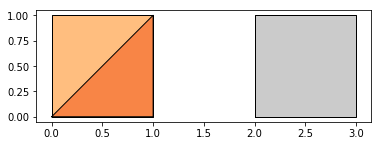GeoPandas还实现了可以读取fiona识别的任何数据格式的替代构造函数。读取纽约市行政区文件：

In :
```# boros = GeoDataFrame.from_file('nybb.shp')
# boros.set_index('BoroCode', inplace=True)
# boros.sort()
```
In :
```# boros['geometry'].convex_hull

```

In :
```from shapely.geometry import Point
import numpy as np
xmin, xmax, ymin, ymax = 900000, 1080000, 120000, 280000
xc = (xmax - xmin) * np.random.random(2000) + xmin
yc = (ymax - ymin) * np.random.random(2000) + ymin
pts = GeoSeries([Point(x, y) for x, y in zip(xc, yc)])
```

In :
```circles = pts.buffer(2000)
```

In :
```mp = circles.unary_union
```

In :
```# holes = boros['geometry'].intersection(mp)
```

In :
```# boros_with_holes = boros['geometry'].difference(mp)
```

In :
```# holes.area / boros.geometry.area
```

### 六、使用覆盖设置操作

（熟悉shapely库的用户的请注意：overlay可以被认为是提供标准形状操作的版本，对于处理两个GeoSeries应用集合操作的复杂性来讲，标准的形状集合操作也可以作为GeoSeries方法。）

#### 1、不同的Overlay操作

In :
```from shapely.geometry import Polygon

polys1 = gpd.GeoSeries([Polygon([(0,0), (2,0), (2,2), (0,2)]),
Polygon([(2,2), (4,2), (4,4), (2,4)])])

polys2 = gpd.GeoSeries([Polygon([(1,1), (3,1), (3,3), (1,3)]),
Polygon([(3,3), (5,3), (5,5), (3,5)])])

df1 = gpd.GeoDataFrame({'geometry': polys1, 'df1':[1,2]})
df2 = gpd.GeoDataFrame({'geometry': polys2, 'df2':[1,2]})
```

In :
```ax = df1.plot(color='red');

df2.plot(ax=ax, color='green');
```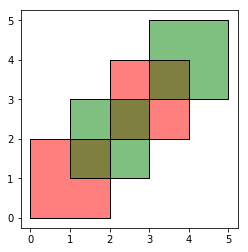In :
```res_union = gpd.overlay(df1, df2, how='union')

res_union
```
Out:
df1 df2 geometry
0 1.0 NaN POLYGON ((2 1, 2 0, 0 0, 0 2, 1 2, 1 1, 2 1))
1 1.0 1.0 POLYGON ((2 1, 1 1, 1 2, 2 2, 2 1))
2 NaN 1.0 POLYGON ((2 1, 2 2, 3 2, 3 1, 2 1))
3 NaN 1.0 POLYGON ((2 2, 1 2, 1 3, 2 3, 2 2))
4 2.0 NaN POLYGON ((3 2, 3 3, 4 3, 4 2, 3 2))
5 2.0 1.0 POLYGON ((3 3, 3 2, 2 2, 2 3, 3 3))
6 2.0 NaN POLYGON ((3 3, 2 3, 2 4, 3 4, 3 3))
7 NaN 2.0 POLYGON ((4 3, 4 4, 3 4, 3 5, 5 5, 5 3, 4 3))
8 2.0 2.0 POLYGON ((3 4, 4 4, 4 3, 3 3, 3 4))
In :
```ax = res_union.plot()

df1.plot(ax=ax, facecolor='none');

df2.plot(ax=ax, facecolor='none');
```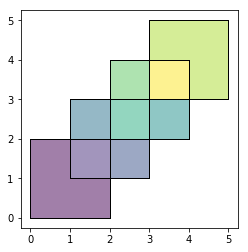In :
```res_intersection = gpd.overlay(df1, df2, how='intersection')

res_intersection
```
Out:
df1 df2 geometry
0 1 1 POLYGON ((2 1, 1 1, 1 2, 2 2, 2 1))
1 2 1 POLYGON ((3 3, 3 2, 2 2, 2 3, 3 3))
2 2 2 POLYGON ((3 4, 4 4, 4 3, 3 3, 3 4))
In :
```ax = res_intersection.plot()

df1.plot(ax=ax, facecolor='none');

df2.plot(ax=ax, facecolor='none');
```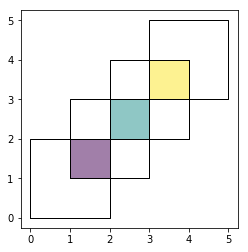how ='symmetric_difference'与'intersection'相反，返回的几何只是GeoDataFrames其中之一的一部分，并不是两者的一部分：

In :
```res_symdiff = gpd.overlay(df1, df2, how='symmetric_difference')

res_symdiff
```
Out:
df1 df2 geometry
0 1.0 NaN POLYGON ((2 1, 2 0, 0 0, 0 2, 1 2, 1 1, 2 1))
1 NaN 1.0 POLYGON ((2 1, 2 2, 3 2, 3 1, 2 1))
2 NaN 1.0 POLYGON ((2 2, 1 2, 1 3, 2 3, 2 2))
3 2.0 NaN POLYGON ((3 2, 3 3, 4 3, 4 2, 3 2))
4 2.0 NaN POLYGON ((3 3, 2 3, 2 4, 3 4, 3 3))
5 NaN 2.0 POLYGON ((4 3, 4 4, 3 4, 3 5, 5 5, 5 3, 4 3))
In :
```ax = res_symdiff.plot()

df1.plot(ax=ax, facecolor='none');

df2.plot(ax=ax, facecolor='none');
```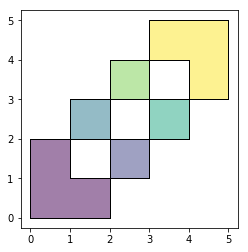In :
```res_difference = gpd.overlay(df1, df2, how='difference')

res_difference
```
Out:
df1 df2 geometry
0 1 None POLYGON ((2 1, 2 0, 0 0, 0 2, 1 2, 1 1, 2 1))
1 2 None POLYGON ((3 2, 3 3, 4 3, 4 2, 3 2))
2 2 None POLYGON ((3 3, 2 3, 2 4, 3 4, 3 3))
In :
```ax = res_difference.plot()

df1.plot(ax=ax, facecolor='none');

df2.plot(ax=ax, facecolor='none');
```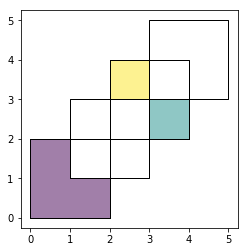In :
```res_identity = gpd.overlay(df1, df2, how='identity')

res_identity

```
Out:
df1 df2 geometry
0 1 NaN POLYGON ((2 1, 2 0, 0 0, 0 2, 1 2, 1 1, 2 1))
1 1 1.0 POLYGON ((2 1, 1 1, 1 2, 2 2, 2 1))
2 2 NaN POLYGON ((3 2, 3 3, 4 3, 4 2, 3 2))
3 2 1.0 POLYGON ((3 3, 3 2, 2 2, 2 3, 3 3))
4 2 NaN POLYGON ((3 3, 2 3, 2 4, 3 4, 3 3))
5 2 2.0 POLYGON ((3 4, 4 4, 4 3, 3 3, 3 4))
In :
```ax = res_identity.plot()

df1.plot(ax=ax, facecolor='none');

df2.plot(ax=ax, facecolor='none');
```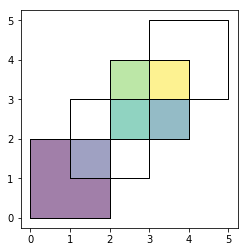#### 2、覆盖国家的示例

In :
```world = gpd.read_file(gpd.datasets.get_path('naturalearth_lowres'))

# Select South Amarica and some columns
countries = world[world['continent'] == "South America"]

countries = countries[['geometry', 'name']]

# Project to crs that uses meters as distance measure
countries = countries.to_crs('+init=epsg:3395')

capitals = capitals.to_crs('+init=epsg:3395')
```

In :
```# Look at countries:
countries.plot();

# Now buffer cities to find area within 500km.
# Check CRS -- World Mercator, units of meters.
capitals.crs
'+init=epsg:3395'

# make 500km buffer
capitals['geometry']= capitals.buffer(500000)

capitals.plot();
```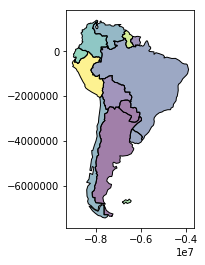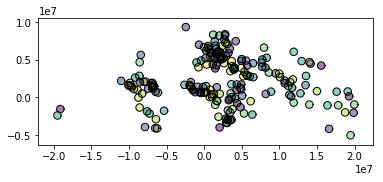In :
```country_cores = gpd.overlay(countries, capitals, how='intersection')
```
In :
```country_cores.plot();
```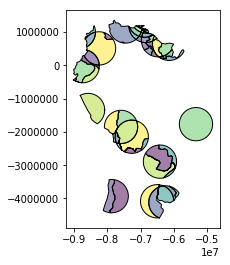In :
```country_peripheries = gpd.overlay(countries, capitals, how='difference')
```
In :
```country_peripheries.plot();
```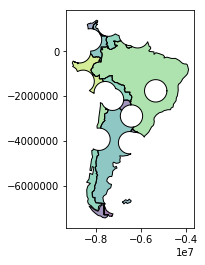### 七、溶解聚集

dissolve可以做三件事情：（a）它将给定组中的所有几何图形，并一起溶解成单个几何特征（使用unary_union方法）；（b）它聚合组中的所有数据行，使用的是groupby.aggregate（）方法；（c）将以上这两种方法结合。

#### 1、dissolve示例

In :
```world = gpd.read_file(gpd.datasets.get_path('naturalearth_lowres'))

world = world[['continent', 'geometry']]

continents = world.dissolve(by='continent')

continents.plot();

```
Out:
geometry
continent
Africa (POLYGON ((49.54351891459575 -12.4698328589405...
Antarctica (POLYGON ((-159.2081835601977 -79.497059421708...
Asia (POLYGON ((120.7156087586305 -10.2395813940878...
Europe (POLYGON ((-52.55642473001839 2.50470530843705...
North America (POLYGON ((-61.68000000000001 10.76, -61.105 1...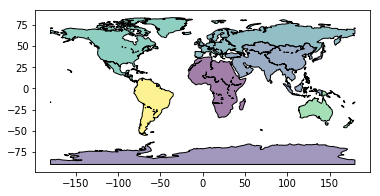In [ ]:
```
```

### 合并数据

In :
```%matplotlib inline
import geopandas as gpd

# For attribute join
country_shapes = world[['geometry', 'iso_a3']]

country_names = world[['name', 'iso_a3']]

# For spatial join
countries = world[['geometry', 'name']]

countries = countries.rename(columns={'name':'country'})
```

#### 属性连接

In :
```#country_shapes` is GeoDataFrame with country shapes and iso codes

```
Out:
geometry iso_a3
0 POLYGON ((61.21081709172574 35.65007233330923,... AFG
1 (POLYGON ((16.32652835456705 -5.87747039146621... AGO
2 POLYGON ((20.59024743010491 41.85540416113361,... ALB
3 POLYGON ((51.57951867046327 24.24549713795111,... ARE
4 (POLYGON ((-65.50000000000003 -55.199999999999... ARG
In :
```country_names.head()
```
Out:
name iso_a3
0 Afghanistan AFG
1 Angola AGO
2 Albania ALB
3 United Arab Emirates ARE
4 Argentina ARG
In :
```country_shapes = country_shapes.merge(country_names, on='iso_a3')

```
Out:
geometry iso_a3 name
0 POLYGON ((61.21081709172574 35.65007233330923,... AFG Afghanistan
1 (POLYGON ((16.32652835456705 -5.87747039146621... AGO Angola
2 POLYGON ((20.59024743010491 41.85540416113361,... ALB Albania
3 POLYGON ((51.57951867046327 24.24549713795111,... ARE United Arab Emirates
4 (POLYGON ((-65.50000000000003 -55.199999999999... ARG Argentina

#### 空间连接

In :
```# One GeoDataFrame of countries, one of Cities.
# Want to merge so we can get each city's country.
```
Out:
geometry country
0 POLYGON ((61.21081709172574 35.65007233330923,... Afghanistan
1 (POLYGON ((16.32652835456705 -5.87747039146621... Angola
2 POLYGON ((20.59024743010491 41.85540416113361,... Albania
3 POLYGON ((51.57951867046327 24.24549713795111,... United Arab Emirates
4 (POLYGON ((-65.50000000000003 -55.199999999999... Argentina
In :
```cities.head()
```
Out:
geometry name
0 POINT (12.45338654497177 41.90328217996012) Vatican City
1 POINT (12.44177015780014 43.936095834768) San Marino
2 POINT (9.516669472907267 47.13372377429357) Vaduz
3 POINT (6.130002806227083 49.61166037912108) Luxembourg
4 POINT (158.1499743237623 6.916643696007725) Palikir
In :
```cities_with_country = gpd.sjoin(cities, countries, how="inner", op='intersects')

```
Out:
geometry name index_right country
0 POINT (12.45338654497177 41.90328217996012) Vatican City 79 Italy
1 POINT (12.44177015780014 43.936095834768) San Marino 79 Italy
192 POINT (12.481312562874 41.89790148509894) Rome 79 Italy
2 POINT (9.516669472907267 47.13372377429357) Vaduz 9 Austria
184 POINT (16.36469309674374 48.20196113681686) Vienna 9 Austria

### 其他

#### 地理编码

``````geopandas.geocode.geocode(strings, provider='googlev3', **kwargs)

``````

### 数据结构

#### GeoSeries

GeoPandas主要实现两个主要的数据结构，GeoSeries和GeoDataFrame，分别是pandas系列和DataFrame的子类。

GeoSeries本质上是一种向量，向量中的每个条目都对应观察的一组形状。条目可以由一个形状（如单个多边形）组成或由多个形状组成，这些形状被认为是一个观察（例如构成夏威夷州或印度尼西亚等国家的许多多边形）。

geopandas有三个基本类的几何对象（实际上是形状对象）：

• 点/点集合
• 线/线集合
• 多边形/多边形集合

#### ①、属性和方法概述

GeoSeries类几乎实现了Shapely对象的所有属性和方法。当应用于GeoSeries时，它们将以元素方式应用于GeoSeries中的所有几何。在两个GeoSeries之间可以应用二进制操作，在这种情况下，操作是按元素进行的。这两个系列将通过匹配索引完成对齐。二进制操作也可以应用于单个几何，在这种情况下，对具有该几何系列的每个元素执行操作。在任一情况下，将酌情返回一个Series或一个GeoSeries。

#### ②、属性

• area：形状面积（投影单位）
• bounds：每个轴的每个形状的最大与最小坐标元组
• total_bounds：整个GeoSeries的每个轴上的最大与最小坐标元组
• geom_type：几何类型。
• is_valid：测试坐标是否形成合理的几何形状。

#### ③、基本方法

• distance（other）：返回每个条目到其他条目最小距离的序列
• centroid：返回质心的GeoSeries
• representative_point（）：返回位于每个几何中点的GeoSeries。它不返回质心。
• to_crs（）：更改坐标参考系。
• plot（）：绘制GeoSeries。

#### ④、关系测试

• geom_almost_equals（other）：形状几乎和其他的一样（由于浮点精度问题导致的形状略有不同，效果还是不错的）
• contains（other）：其他包含的形状
• intersects（other）：其他相交的形状

### 数据结构：GeoDataFrame

GeoDataFrame是一个包含GeoSeries的表格数据结构。

GeoDataFrame最重要的属性是它总是具有一个保存特殊状态的GeoSeries列。此GeoSeries称为GeoDataFrame的“几何”。当空间方法应用于GeoDataFrame（或调用类似区域的空间属性）时，此命令将始终作用于“几何”列。

“geometry”列可通过geometry属性（gdf.geometry）访问，并且可以通过键入gdf.geometry.name的方法找到geometry列名称。

GeoDataFrame还可以包含具有几何（形状）对象的其他列，但每次只能有一个列作为活动式几何。若更改活动式几何列，可使用set_geometry方法。

In :
```%matplotlib inline
import geopandas as gpd

```
Out:
continent gdp_md_est geometry iso_a3 name pop_est
0 Asia 22270.0 POLYGON ((61.21081709172574 35.65007233330923,... AFG Afghanistan 28400000.0
1 Africa 110300.0 (POLYGON ((16.32652835456705 -5.87747039146621... AGO Angola 12799293.0
2 Europe 21810.0 POLYGON ((20.59024743010491 41.85540416113361,... ALB Albania 3639453.0
3 Asia 184300.0 POLYGON ((51.57951867046327 24.24549713795111,... ARE United Arab Emirates 4798491.0
4 South America 573900.0 (POLYGON ((-65.50000000000003 -55.199999999999... ARG Argentina 40913584.0
In :
```world.plot();
```In :
```world.geometry.name
```
Out:
`'geometry'`

In :
```world = world.rename(columns={'geometry': 'borders'}).set_geometry('borders')

world.geometry.name
```
Out:
`'borders'`

In :
```world['centroid_column'] = world.centroid

world = world.set_geometry('centroid_column')

world.plot();
```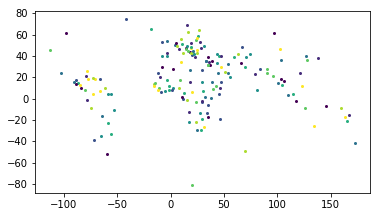``````gdf = gdf.rename(columns={'old_name': 'new_name'}).set_geometry('new_name')

``````

#### ①、属性和方法

GeoSeries所描述的任何属性调用或方法都可以在GeoDataFrame上工作，实际上，它们只是应用于“几何”GeoSeries。

In :
```import helper; helper.info()
```
```页面更新时间： 2019-02-28 19:00:54

Python: 3.5.3
```